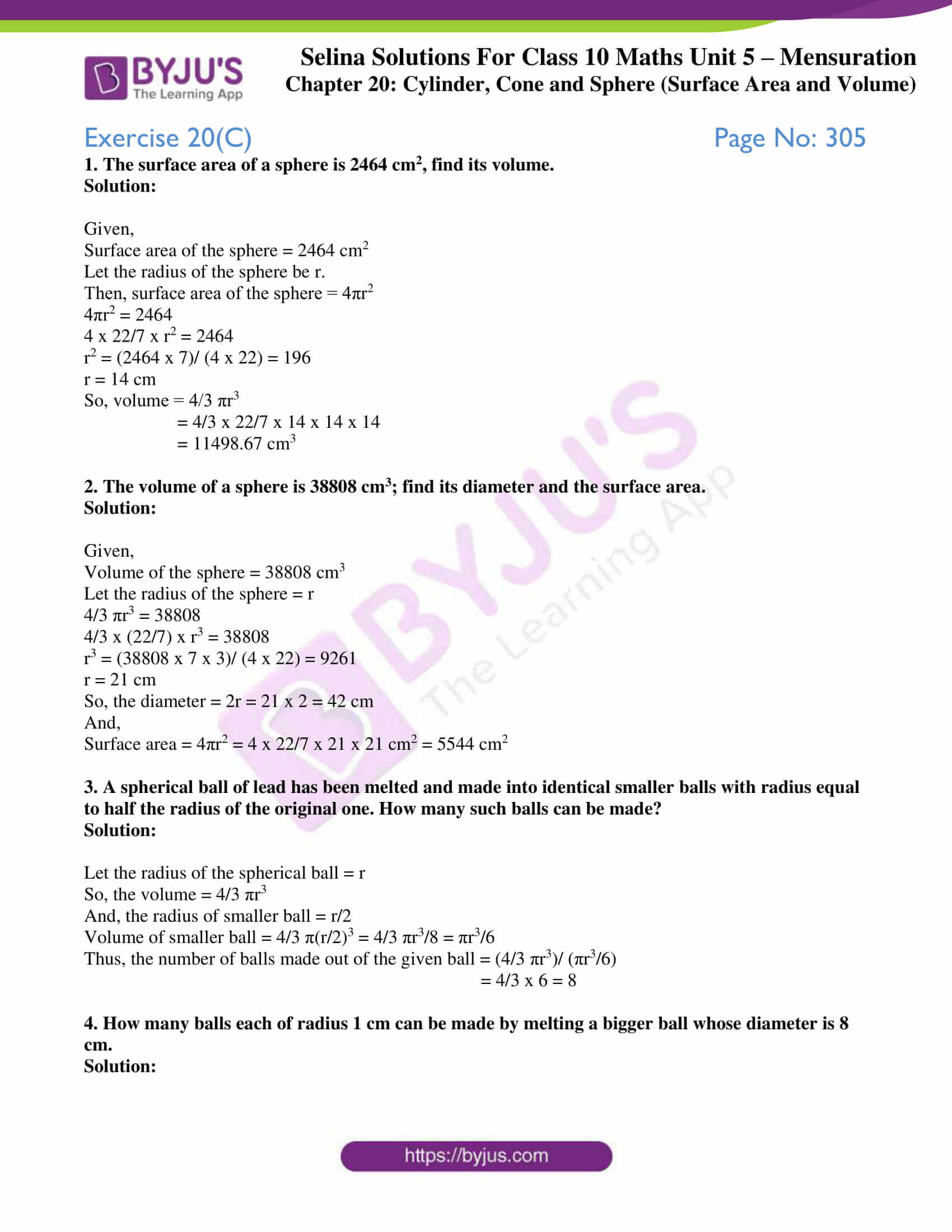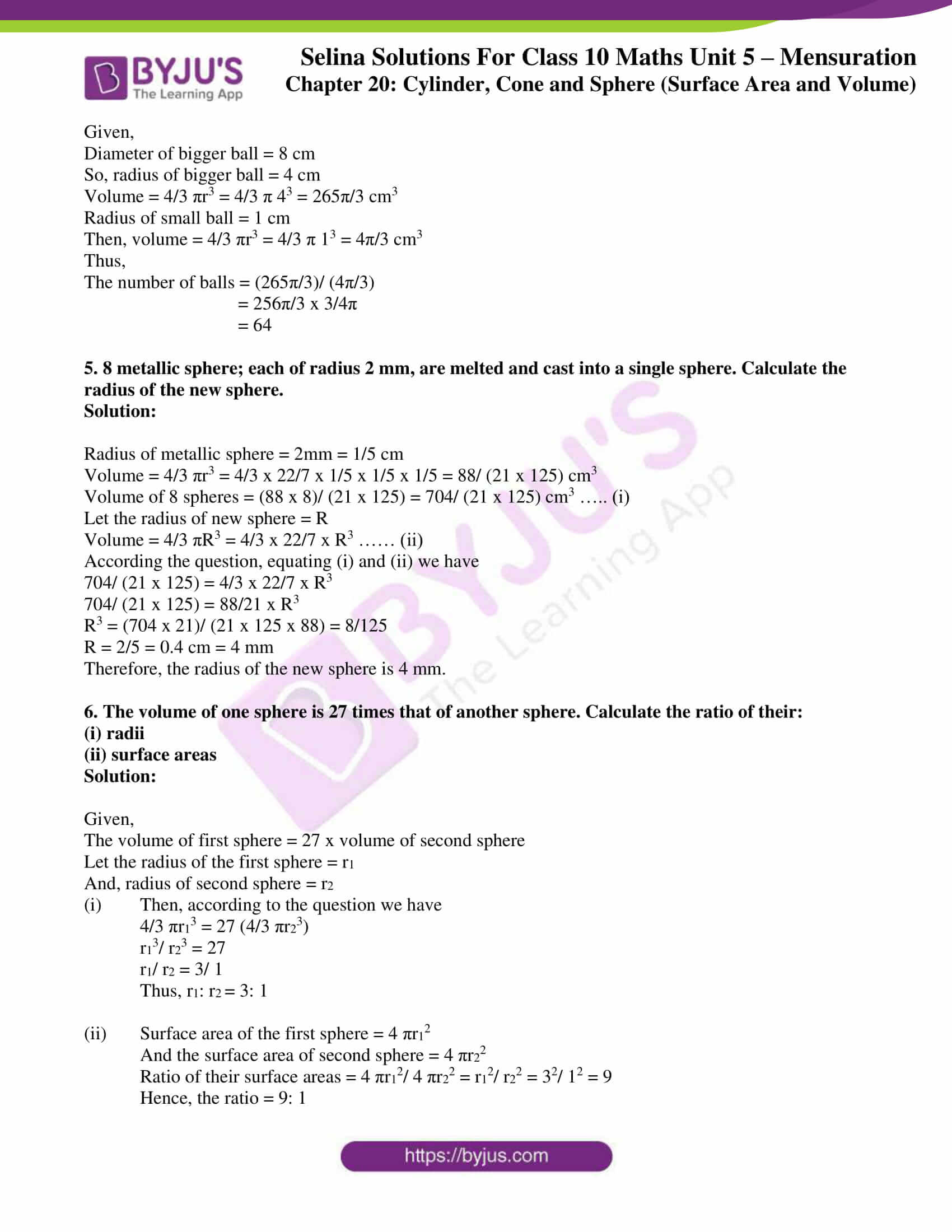# Selina Solutions Concise Maths Class 10 Chapter 20 Cylinder, Cone and Sphere (Surface Area and Volume) Exercise 20(C)

A sphere is a solid obtained on revolving a circle about any diameter of it. So, this exercise deals with problems of finding the surface area and volume of spheres, spherical shells and hemispheres. For students wanting clarity in solving problems, the Selina Solutions for Class 10 Maths is all one needs. The solutions are at par with the latest ICSE marking schemes. Further, the Concise Selina Solutions for Class 10 Maths Chapter 20 Cylinder, Cone and Sphere (Surface Area and Volume) Exercise 20(C) PDF can be accessed in the links given below.

## Selina Solutions Concise Maths Class 10 Chapter 20 Cylinder, Cone and Sphere (Surface Area and Volume) Exercise 20(C) Download PDF### Access Selina Solutions Concise Maths Class 10 Chapter 20 Cylinder, Cone and Sphere (Surface Area and Volume) Exercise 20(C)

1. The surface area of a sphere is 2464 cm2, find its volume.

Solution:

Given,

Surface area of the sphere = 2464 cm2

Let the radius of the sphere be r.

Then, surface area of the sphere = 4πr2

4πr2 = 2464

4 x 22/7 x r2 = 2464

r2 = (2464 x 7)/ (4 x 22) = 196

r = 14 cm

So, volume = 4/3 πr3

= 4/3 x 22/7 x 14 x 14 x 14

= 11498.67 cm3

2. The volume of a sphere is 38808 cm3; find its diameter and the surface area.

Solution:

Given,

Volume of the sphere = 38808 cm3

Let the radius of the sphere = r

4/3 πr3 = 38808

4/3 x (22/7) x r3 = 38808

r3 = (38808 x 7 x 3)/ (4 x 22) = 9261

r = 21 cm

So, the diameter = 2r = 21 x 2 = 42 cm

And,

Surface area = 4πr2 = 4 x 22/7 x 21 x 21 cm2 = 5544 cm2

3. A spherical ball of lead has been melted and made into identical smaller balls with radius equal to half the radius of the original one. How many such balls can be made?

Solution:

Let the radius of the spherical ball = r

So, the volume = 4/3 πr3

And, the radius of smaller ball = r/2

Volume of smaller ball = 4/3 π(r/2)3 = 4/3 πr3/8 = πr3/6

Thus, the number of balls made out of the given ball = (4/3 πr3)/ (πr3/6)

= 4/3 x 6 = 8

4. How many balls each of radius 1 cm can be made by melting a bigger ball whose diameter is 8 cm.

Solution:

Given,

Diameter of bigger ball = 8 cm

So, radius of bigger ball = 4 cm

Volume = 4/3 πr3 = 4/3 π 43 = 265π/3 cm3

Radius of small ball = 1 cm

Then, volume = 4/3 πr3 = 4/3 π 13 = 4π/3 cm3

Thus,

The number of balls = (265π/3)/ (4π/3)

= 256π/3 x 3/4π

= 64

5. 8 metallic sphere; each of radius 2 mm, are melted and cast into a single sphere. Calculate the radius of the new sphere.

Solution:

Radius of metallic sphere = 2mm = 1/5 cm

Volume = 4/3 πr3 = 4/3 x 22/7 x 1/5 x 1/5 x 1/5 = 88/ (21 x 125) cm3

Volume of 8 spheres = (88 x 8)/ (21 x 125) = 704/ (21 x 125) cm3 ….. (i)

Let the radius of new sphere = R

Volume = 4/3 πR3 = 4/3 x 22/7 x R3 …… (ii)

According the question, equating (i) and (ii) we have

704/ (21 x 125) = 4/3 x 22/7 x R3

704/ (21 x 125) = 88/21 x R3

R3 = (704 x 21)/ (21 x 125 x 88) = 8/125

R = 2/5 = 0.4 cm = 4 mm

Therefore, the radius of the new sphere is 4 mm.

6. The volume of one sphere is 27 times that of another sphere. Calculate the ratio of their:

(ii) surface areas

Solution:

Given,

The volume of first sphere = 27 x volume of second sphere

Let the radius of the first sphere = r1

And, radius of second sphere = r2

(i) Then, according to the question we have

4/3 πr13 = 27 (4/3 πr23)

r13/ r23 = 27

r1/ r2 = 3/ 1

Thus, r1: r2 = 3: 1

(ii) Surface area of the first sphere = 4 πr12

And the surface area of second sphere = 4 πr22

Ratio of their surface areas = 4 πr12/ 4 πr22 = r12/ r22 = 32/ 12 = 9

Hence, the ratio = 9: 1